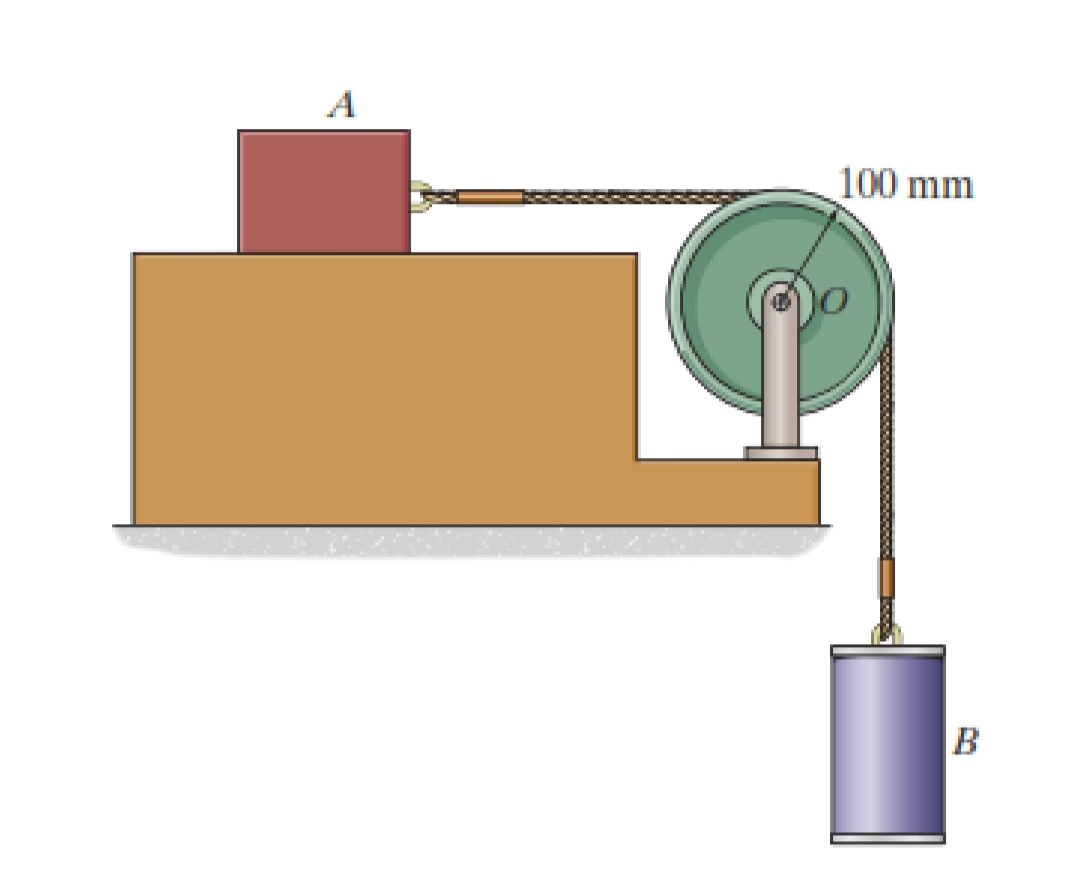## Calculate the angular acceleration of the pulley.

The 15-kg block A and 25-kg cylinder B are connected by the light cord that passes over a 5-kg pulley with IG = 0.025 kg.m2. If the system is released from rest, (with coefficient of friction between the block and ground of 0.3) calculate the angular acceleration of the pulley.in progress